# Gas/Oil Interfacial Tension

Jump to: navigation, search

## Gas/Oil Interfacial Tension

Gas/Oil Interfacial Tension correlation was published by Baker and Swerdloff in 1956.

## Math and Physics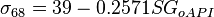$\sigma_{68} =39 - 0.2571 SG_{oAPI}$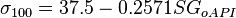$\sigma_{100} =37.5 - 0.2571 SG_{oAPI}$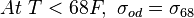$At\ T<68F,\ \sigma_{od}=\sigma_{68}$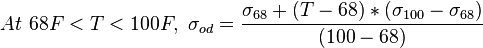$At\ 68F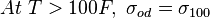$At\ T>100F,\ \sigma_{od}=\sigma_{100}$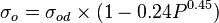$\sigma_{o}=\sigma_{od} \times (1-0.24P^{0.45})$

The interfacial tension becomes zero at miscibility pressure. 1 dyne/cm is used for calculations, then it becomes less than 1.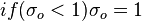$if (\sigma_{o}<1) \sigma_{o}=1$

## Nomenclature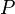$P$ = pressure, psia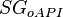$SG_{oAPI}$ = oil specific gravity, °API$\sigma_{o}$ = gas/oil interfacial tension, dynes/cm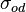$\sigma_{od}$ = gas/oil interfacial tension for dead oil, dynes/cm$T$ = temperature, °F

## See also

Gas/Oil Interfacial Tension
Gas/Water Interfacial Tension